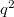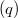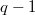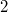﻿

#### Abstract

Some new results on symplectic translation planes  are given using their representation by spread sets of symmetric matrices. We provide a general construction of symplectic planes of even order and then consider the special case of planes of order$q^2$ with kernel containing$\GF(q)$, stressing the role of Brown's theorem on ovoids containing a conic section. In particular we provide a criterion for a symplectic plane of even order$q^2$  with  kernel containing$\GF(q)$ to be desarguesian. As a consequence we prove  that a symplectic plane of even order$q^2$ with  kernel containing$\GF(q)$ and  admitting an affine homology of order$q-1$ or a Baer involution fixing a totally isotropic$2$-subspace  is desarguesian. Finally a short proof that symplectic semifield planes of even order$q^2$ with  kernel containing$\GF(q)$ are desarguesian is given.

DOI Code: 10.1285/i15900932v29n1supplp153

Keywords:
translation plane; symplectic spread; line-oval; affine homology; Baer involution

Full Text: PDF